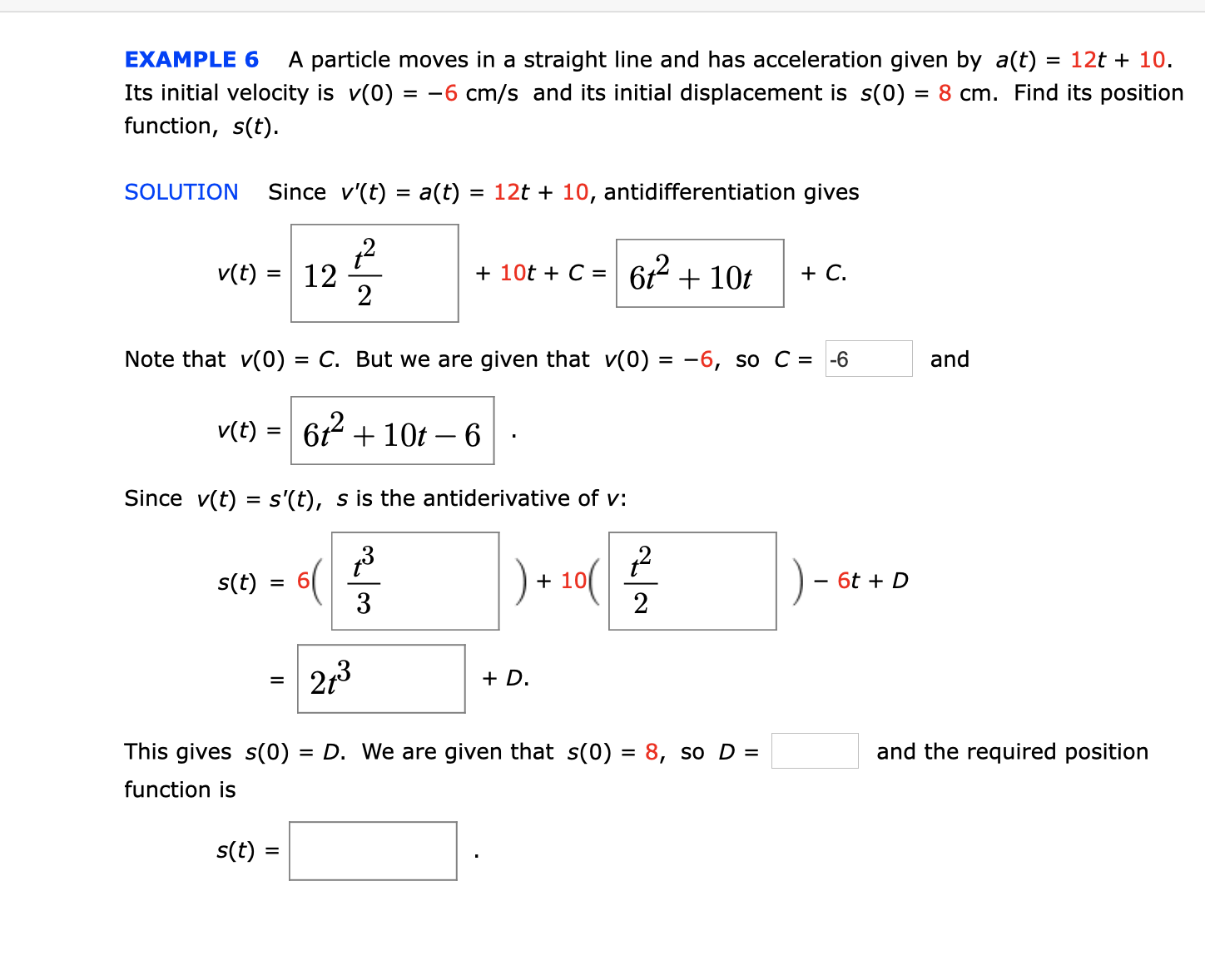# EXAMPLE 6A particle moves in a straight line and has acceleration given by a(t) = 12t + 10.Its initial velocity is v(0) = -6 cm/s and its initial displacement is s(0) = 8 cm. Find its positionfunction, s(t)= 12t 10, antidifferentiation givesSince v'(t) a(t)SOLUTION6t2+10tv(t)+C12210t C =Note that v(0) = C. But we are given that v(0) = -6, so C = -6and6t210t-6v(t)=s'(t), s is the antiderivative of v:Since v(t)6t D+10s(t)622p3D.This gives s(0)and the required position= D. We are given that s(0) = 8, so D =function iss(t)=

Question
316 viewshelp_outlineImage TranscriptioncloseEXAMPLE 6 A particle moves in a straight line and has acceleration given by a(t) = 12t + 10. Its initial velocity is v(0) = -6 cm/s and its initial displacement is s(0) = 8 cm. Find its position function, s(t) = 12t 10, antidifferentiation gives Since v'(t) a(t) SOLUTION 6t2+10t v(t) +C 12 2 10t C = Note that v(0) = C. But we are given that v(0) = -6, so C = -6 and 6t210t-6 v(t) = s'(t), s is the antiderivative of v: Since v(t) 6t D +10 s(t) 6 2 2p3 D. This gives s(0) and the required position = D. We are given that s(0) = 8, so D = function is s(t) = fullscreen
check_circle

Step 1

To find the position function, s(t).

Wh...

### Want to see the full answer?

See Solution

#### Want to see this answer and more?

Solutions are written by subject experts who are available 24/7. Questions are typically answered within 1 hour.*

See Solution
*Response times may vary by subject and question.
Tagged in

### Other